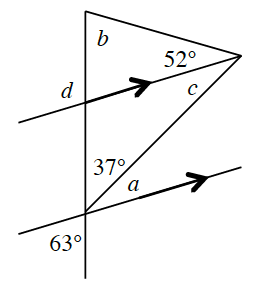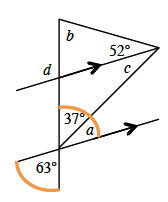### Home > GC > Chapter 7 > Lesson 7.1.1 > Problem7-7

7-7.Use the relationships in the diagram at right to find the values of each variable. Name which geometric relationships you used.

Refer to the Math Notes box in Lesson 2.1.4 if you need help establishing any angle pair relationship.

Since the highlighted angles are vertical angles, and vertical angles are equal, $37°+a=63°$.

$∠c$ is an alternate interior angle to $∠a$. Recall that alternate interior angles are equal.

Use the Triangle Angle Sum Theorem to calculate $b$: $37°+c+52+b=180°$.

To find $m∠d$, notice that it is the exterior angle in the small triangle that contains $∠b$ and $52°$. What relationship do those angles have?